#Nonhomogeneous Linear EquationsConsider the nonhomogeneous linear equation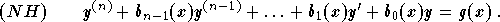We have seen that the general solution is given by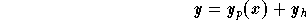,

where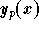is a particular solution and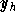is the general solution of the associated homogeneous equation. We will not discuss the case of non-constant coefficients. Therefore, we will restrict ourself only to the following type of equation: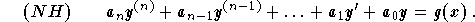Using the previous section, we will discuss how to find the general solution of the associated homogeneous equation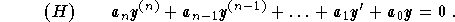Therefore, the only remaining obstacle is to find a particular solution to (NH). In the second order differential equations case, we learned the two methods: Undetermined Coefficients Method and the Variation of Parameters. These two methods are still valid in the general case, but the second one is very hard to carry.[Differential Equations] [First Order D.E.] [Second Order D.E.]
[Geometry] [Algebra] [Trigonometry ]
[Calculus] [Complex Variables] [Matrix Algebra]S.O.S MATHematics home page

Do you need more help? Please post your question on our S.O.S. Mathematics CyberBoard.Author: Mohamed Amine Khamsi